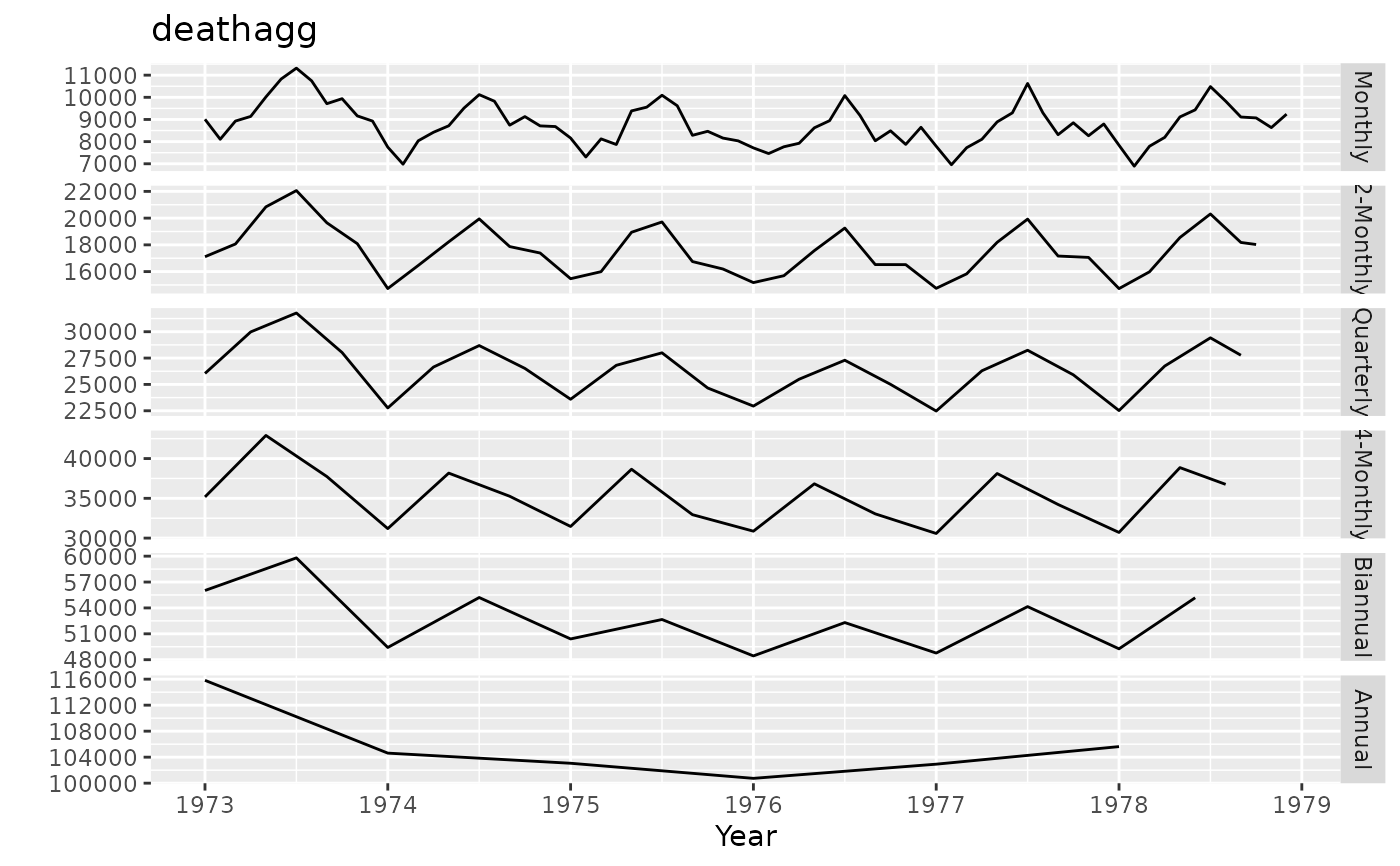Plots all temporal aggregations of a time series

# S3 method for tsaggregates
plot(x, series = "all", ...)

# S3 method for tsaggregates
autoplot(object, series = "all", ...)

## Arguments

x tsaggregates object, produced by tsaggregates. The indexes of the series to plot. By default, all series are plotted. Other arguments passed to plot.ts or autoplot.ts. tsaggregates object, produced by tsaggregates.

## Examples

deathagg <- tsaggregates(USAccDeaths)
plot(deathagg, series=c(1,2,4,6))library(ggplot2)
autoplot(deathagg)